# Colouring the square of a planar graph

 Importance: Medium ✭✭
 Author(s): Wegner
 Subject: Graph Theory » Coloring » » Vertex coloring
 Keywords:
 Posted by: fhavet on: March 13th, 2013
Conjecture   Letbe a planar graph of maximum degree. The chromatic number of its square is
\item at most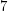if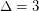, \item at mostif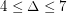, \item at most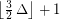if.

The square of a graphis the graph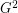on the same set of vertices, in which two vertices are adjacent when their distance inis at most 2.

Wegner [W] also gave examples showing that these bounds would be tight. For, they are the following.For, the examples are planar graphs onwith maximum degreewhose square is a complete graph.

This conjecture has also been generalized to the list chromatic number.

Conjecture   Letbe a planar graph of maximum degree. The list chromatic number of its square is
\item at mostif, \item at mostif, \item at mostif.

Cranston and Kim [CK] showed that the square of every connected graph (non necessarily planar) which is subcubic (i.e., with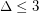) is 8-choosable, except for the Petersen graph. However, the 7-choosability of the square of subcubic planar graphs is still open.

Havet et al. [HHMR] proved the conjecture asymptotically:

Theorem   The square of every planar graphof maximum degreehas list chromatic number at most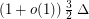.

In fact, they proved this results for more general classes of graph. This led them to pose the following problem.

Problem   Is it true that for every minor-closed familyof graphs (withnot the set of all graphs), we havefor all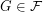?

## Bibliography

[HHMR] F. Havet, J. van den Heuvel, C. McDiarmid, and B. Reed. List Colouring Squares of Planar Graphs. Research Report RR-6586, INRIA, July 2008.

[CK] D. W. Cranston and S.-J. Kim. List-coloring the square of a subcubic graph, J. Graph Theory, 57(1):65--87, 2008.

*[W] G. Wegner. Graphs with given diameter and a coloring problem. Technical report, 1977.

* indicates original appearance(s) of problem.